Checkout JEE MAINS 2022 Question Paper Analysis : Checkout JEE MAINS 2022 Question Paper Analysis :

# Important 5 Marks Questions for CBSE 12th Chemistry Exam

Class 12 chemistry important 5 mark questions with answers are provided here. These important questions are based on CBSE board curriculum and correspond to the most recent Class 12 chemistry syllabus. By practicing these Class 12 important 5 mark questions, students will be able to quickly review all of the ideas covered in the chapter and prepare for the Class 12 Annual examinations as well as other entrance exams such as NEET and JEE.

Download Class 12 Chemistry Important 5 Mark Questions with Answers PDF by clicking on the button below.

## Class 12 Chemistry Important 5 Mark Questions with Answers

Question 1:

(a) Why do transition elements show variable oxidation states?

1. Name the elements showing a maximum number of oxidation states among the first series of transition metals from Sc (Z = 21) to Zn (Z=30)
2. Name the element which shows only +3 oxidation state

(b) What is lanthanide contraction? Name an important alloy that contains some of the lanthanoid metals

(a) Transition elements are elements with partially filled d orbitals (also known as transition metals). Transition elements are defined by IUPAC as elements with a partially filled d subshell or elements capable of forming stable cations with an incompletely filled d orbital. The transition element’s electronic configuration is (n-1)d5 ns1 or (n-1)d10 ns1.

Since their valence electrons are in two different sets of orbitals, (n-1)d and ns, these elements have variable oxidation states. The energy difference between these orbitals is so small that both energy levels can be used to form bonds. As a result, transition elements have variable oxidation states.

1. Mn(Z-25) has the highest number of unpaired electrons in the d-subshell, and it shows a high oxidation state(+7).

2. Scandium (Sc) only exhibits a +3 oxidation state in these series.

(b) The steady decrease in atomic and ionic radii (with the same charge) with increasing atomic number as we move from lanthanum to lutetium is referred to as lanthanoid contraction. Mischmetal is an important lanthanoid metal alloy that contains 95%lanthanide metal and 5% Fe, as well as traces of S, C, Ca, and Al. It is used to make bullets, shells, and lighter flint in Mg-based alloys.

Question 2:

(a) Given reasons for the following:

1. Mn3+ is a good oxidizing agent.
2. E°M2+/M values are not regular for first-row transition metals(3d series)
3. Although ‘F’ is more electronegative than ‘O’, the highest Mn fluoride is MnF4 whereas the highest oxide is Mn2O7.

(b) Complete the following equation:

1. $$\begin{array}{l}2CrO_{4}^{2-}+2H^{+}\to \end{array}$$
2. $$\begin{array}{l}KMnO_{4}\xrightarrow[]{heat}\end{array}$$

(a)

1. A good oxidising agent reduces itself, which means it gains electrons from other substances. To achieve a stable electronic configuration, it tends to gain one more electron. Mn3+ is a good oxidising agent because the +2 oxidation state of Mn is more stable than the +3 oxidation state. Mn +3 has been reduced to Mn2+.

2. E°M2+/M values are not regular for first-row transition metals(3d series) because the values of first Ionization energy and second Ionization energy are not consistent for transition metals in the first row of the 3d series.

3. Fluorine can form a single bond while oxygen can form multiple bonds.

(b)

1.

$$\begin{array}{l}2CrO_{4}^{2-}+2H^{+}\to Cr_{2}O_{7}^{2-}+H_{2}O\end{array}$$

2.

$$\begin{array}{l}KMnO_{4}\xrightarrow[]{heat}K_{2}MnO_{4}+MnO_{2}+O_{2}\end{array}$$

Question 3:

(a) Define the following terms:

1. Ideal solution
2. Azeotrope
3. Osmotic pressure

(b) A solution of glucose (C6H12O6) in water is labelled as 10% by weight. What would be the molality of the solution? (Molar mass of glucose = 180 g mol–1)

(a)

1. The ideal solution is one that complies with Raoult’s law. An ideal solution or ideal mixture is a solution in which the enthalpy (ΔHsolution, ΔVsolution, ΔSsolution) of the solution is zero, with the closer to zero the enthalpy of solution, the more “ideal” the solution’s behaviour becomes.

2. A constant boiling mixture, also known as an azeotrope, is a mixture of two or more liquids whose proportions cannot be changed by simple distillation. This is due to the fact that when an azeotrope is boiled, the vapour has the same constituent proportions as the unboiled mixture.

3. Osmotic pressure is the minimum pressure that must be applied to a solution in order to prevent water from passing through a semipermeable membrane. It is also defined as the measure of a solution’s strong tendency to absorb water through osmosis.

(b) Mass of solute w = 10 g Mass of solvent W = 90 g Molar mass of solute M = 180 g mol–1

$$\begin{array}{l}Molality= \frac{w\times 1000}{M\times W}\end{array}$$

$$\begin{array}{l}Molality= \frac{10\times 1000}{90\times 180}=0.1672\approx 0.62 mol kg^{-1}\end{array}$$

Question 4:

(a) How will you convert the following:

1. Propanone to Propan-2-ol
2. Ethanal to 2-hydroxy propanoic acid
3. Toluene to benzoic acid

(b) Give a simple chemical test to distinguish between:

1. Pentan-2-one and Pentan-3-one
2. Ethanal and Propanal

(a)

1. Propanone to Propan-2-ol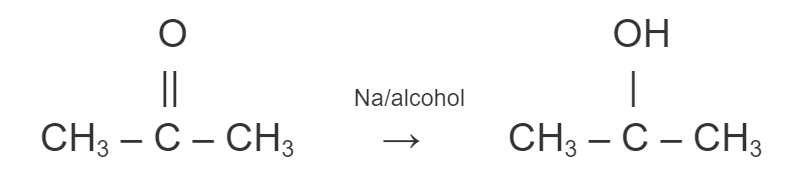2. Ethanal to 2-hydroxy propanoic acid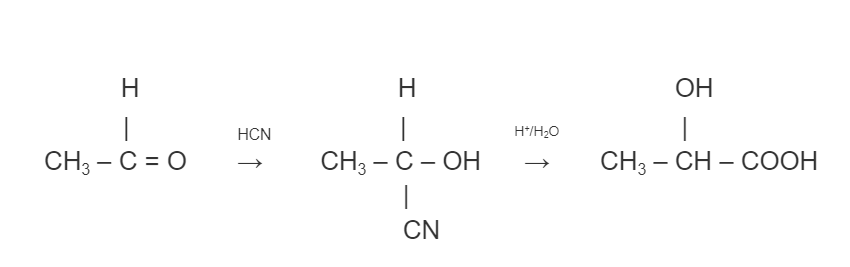(3) Toluene to benzoic acid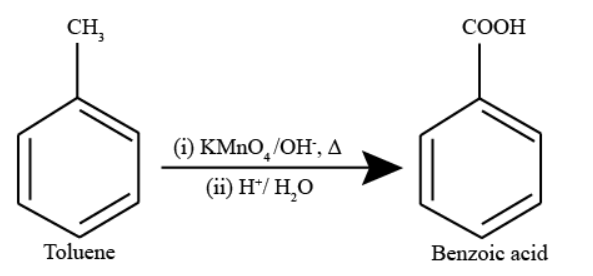(b)

(1) Pentan-2-one and Pentan-3-one

By Iodoform Test

Pentan-2-one being a methyl ketone when treated with NaOl (I2 /NaOH) gives a yellow precipitate of iodoform but pentan-3-one does not.

(2) Ethanal and Propanal

Ethanal when treated with NaOl (I2 /NaOH) gives a yellow precipitate of iodoform but propanal does not.

Question 5: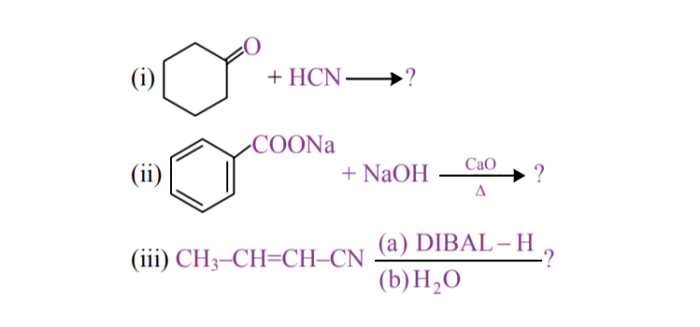(b) Give simple chemical tests to distinguish between the following pairs of compounds:

1. Butanal and Butan-2-one
2. Benzoic acid and Phenol

(a) (i)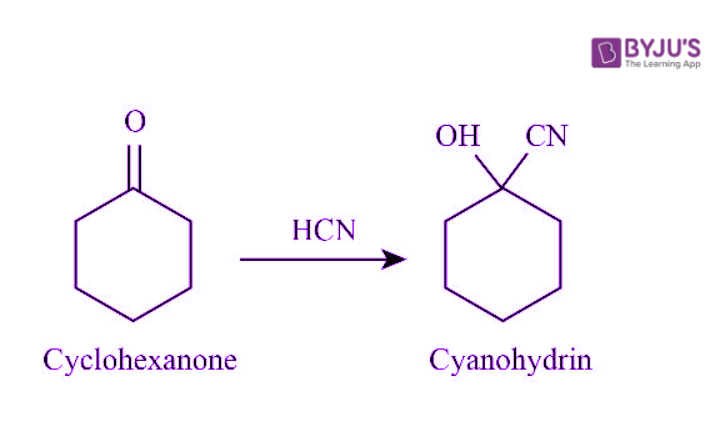(ii)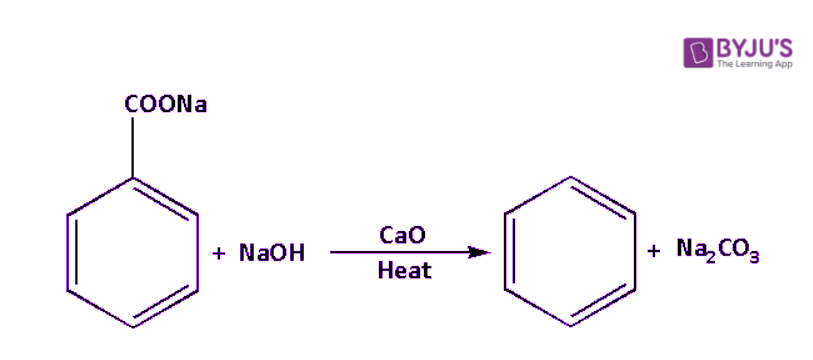(iii)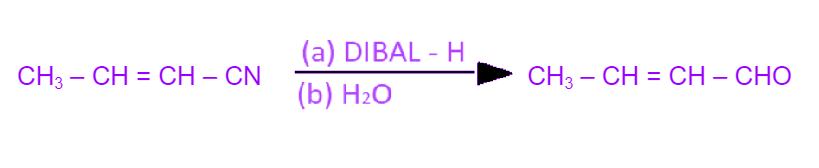(b)

(1) Tollen’s reagent test: Combine both solutions with an ammoniacal solution of silver nitrate (Tollen’s Reagent). Butanal produces a silver mirror, but Butan-2-one does not.

(2) The ferric chloride test can distinguish between benzoic acid and phenol. When neutral FeCl3 is added to both solutions, phenol produces a violet colour while benzoic acid does not.

Question 6:

(a) What type of deviation is shown by a mixture of ethanol and acetone? Give reason.

(b) A solution of glucose (molar mass = 180 g mol–1) in water is labelled as 10% (by mass). What would be the molality and molarity of the solution? (Density of solution = 1.2 g mL–1)

(a) It exhibits a positive deviation. It is because the interaction between acetone and ethanol is weaker than the interaction between ethanol and ethanol.

(b) WB= 10 g, WS = 100 g, WA = 90 g, MB = 180 g/mol and d = 1.2 g/mL

$$\begin{array}{l}M=\frac{Wt%\times density\times 10}{Mol. wt.}\end{array}$$

$$\begin{array}{l}M=\frac{10\times 1.2\times 10}{180}\end{array}$$

= 0.66 M or 0.66 mol/L

$$\begin{array}{l}m=\frac{W_{b}\times 1000}{M_{B}\times W_{A}}\end{array}$$

$$\begin{array}{l}m=\frac{10\times 1000}{180\times 90}\end{array}$$

= 0.61 m or 0.61 mol/kg

Question 7:

(a) Define the following terms:

1. Molarity
2. Molal elevation constant (Kb)

(b) A solution containing 15 g urea (molar mass = 60 g mol–1) per litre of solution in water has the same osmotic pressure (isotonic) as a solution of glucose (molar mass = 190 g mol–1) in water. Calculate the mass of glucose present in one litre of its solution.

(a) 1. The number of moles of solute present in one litre of solvent is defined as a solution’s molarity.

2. Molal elevation constant (Kb ) is defined as the increase in the boiling point of a solution caused by dissolving one mole of a nonvolatile solute in one kilogram of a volatile solvent.

(b) Osmotic pressure π = CRT

where n is the number of moles of solute present in volume V of solvent.
It is given that the solution of urea is isotonic with the solution of glucose, thus-

$$\begin{array}{l}\frac{n_{Glucose}}{V}RT=\frac{n_{Urea}}{V}RT\end{array}$$

$$\begin{array}{l}=Glucose=\frac{15}{60}\times 180=45g\end{array}$$

Hence, the mass of glucose present in one litre of its solution = 45g.

Question 8:

a) Account for the following:

1. Transition metals show variable oxidation states.
2. Zn, Cd and Hg are soft metals.
3. Eº value for the Mn3+/Mn2+ couple is highly positive (+1.57 V) as compared to Cr3+/Cr2+.

b) Write one similarity and one difference between the chemistry of lanthanoid and actinoid elements.

(a)

(1) Due to the relatively small energy difference between the (n-1)d and ns-orbitals, transition elements have variable oxidation states.

(2) The general formula (n-1)d10 ns2 represents the electronic configurations of Zn, Cd, and Hg. The orbitals in these elements are completely filled in both their ground and common oxidation states.

(3) Mn2+ exists in the 3d5 configuration, which is half-filled, and such half-filled configurations provide extra stability to the metal ion. While Mn3+ will exist in a 3d4 configuration, which is less stable than a 3d5 configuration. Because of the 3d3 half filled d orbital, Cr+3 has greater stability than Cr+2. As a result, Cr3+/ Cr2+ is less feasible.

(b) Similarity: The filling of (n-2)f orbitals is involved in lanthanoids and actinoids.

Difference: Lanthanides have a maximum oxidation state of +4, whereas actinides have oxidation states of +3, +4, +5, +6, and +7.

Question 9:

(a) Account for the following:

1. Cl–CH2COOH is a stronger acid than CH3COOH.
2. Carboxylic acids do not give reactions of the carbonyl group.

(b) Write the chemical equations to illustrate the following name reactions:

1. Rosenmund reduction
2. Cannizzaro’s reaction

(c) Out of CH3CH2–CO–CH2–CH3 and CH3CH2–CH2–CO–CH3, which give iodoform test?

(a) 1. Cl, as a result of the -I effect, withdraws electrons from the O-H bond, resulting in a decrease in electron density in the O-H bond. It facilitates the release of H+ by weakening the O-H bond. The +I effect is present in the CH3 group. It increases the electron density in the OH bond, making the release of H+ from acetic acid more difficult than from chloroacetic acid. As a result, CI – CH2COOH is a stronger acid than CH3COOH.

2. Carboxylic acid is a resonance hybrid of the structures I and II shown below.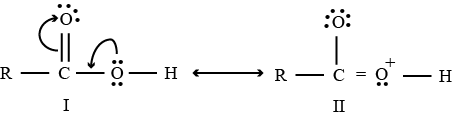A carbonyl group of aldehydes and ketones is also a resonance hybrid of structures III and IV.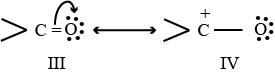The carbonyl carbon of aldehydes and ketones is electrophilic due to structure IV. However, the electrophilic character of carboxyl carbon is reduced due to the contribution of structure (II). As a result, carboxylic acids do not undergo the nucleophilic addition reactions of aldehydes and ketones.

(b) 1. Rosenmund reduction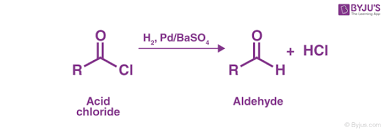2. Cannizzaro’s reaction

Aldehydes that do not contain α-hydrogen, such as HCHO and C 6 H 5 CHO, react with concentrated NaOH solution to form methyl alcohol and formic acid.

2HCHO + NaOH → HCOONa + CH3OH

2C6H5CHO + NaOH → C6H5CH2OH + C6H5COONa

(c) CH3CH2–CH2–CO–CH3 will give the Iodoform test because it has the terminal methyl group.

Question 10:

(i) With reference to structural variability and chemical reactivity, write the difference between lanthanoids and action ions.

(ii) Name of member of the lanthanoid series which is well known to exhibit +4 oxidation state.

(iii) Complete the following equations : MnO4 + 8H+ + 5e

(iv) Out of Mn3+ and Cr3+, which is more paramagnetic and why ?

(atomic nos. : Mn = 25, r = 24)

(i) The following differences exist between lanthanoids and actinoids in terms of structural variability and chemical reactivity:
a) Actinoids are radioactive, whereas lanthanoids are not.
b) The early actinoids have lower ionisation enthalpies than the early lanthanoids. Lanthanoids have an oxidation state ranging from +3 (the most common) to +7, whereas actinoids have an oxidation state ranging from +3 to +7.
c) Actinoids are more reactive than lanthanoids and have more complex magnetic properties.

(ii) Cerium is a lanthanoids element that is known to exhibit the oxidation state of +4.

(iii) MnO4 + 8H+ + 5e → Mn2+ +4 H2O

(iv) Mn2+ is more paramagnetic because it has more number of unpaired electrons than Cr3+.

Question 11:

(a) Write the product(s) in the following reactions :(b) Give simple chemical tests to distinguish between the following pairs of compounds :

1. Butanal and Butan-2-one
2. Benzoic acid and Phenol

(a) (i)(ii)(iii)(b)

(1) Tollen’s reagent test: Combine both solutions with an ammoniacal solution of silver nitrate (Tollen’s Reagent). Butanal produces a silver mirror, but Butan-2-one does not.

(2) The ferric chloride test can distinguish between benzoic acid and phenol. When neutral FeCl3 is added to both solutions, phenol produces a violet colour while benzoic acid does not.

Question 12:

(a) Complete the following equations:

1. Cr2O72– + 2OH
2. MnO4 + 4H+ + 3e

(b) Account for the following :

1. Zn is not considered as a transition element.
2. Transition metals form a larger number of complexes.
3. The Eº value for the Mn3+ / Mn2+ couple is much more positive than that for Cr3+/Cr2+ couple.

(a)

1. Cr2O72– + 2OH → 2CrO42– + H2O
2. MnO4 + 4H+ + 3e → MnO2 + 2H2O

(b)

1. Transition metals are compounds with an incomplete d subshell that form at least one stable ion. Zn is not a transition metal because it only forms Zn2+ ions with all 3d electrons present.
2. Since transition metals have a variable oxidation state, they can form a wide range of complexes.
3. Mn2+ has an outer electronic configuration of 3d4, while Mn2+ has an outer electronic configuration of 3d5. As a result, the conversion is highly advantageous, with a much higher Eº value than a conversion of Cr3+ to Cr2+.

Question 13:

(a) Write the structures of the main products when aniline reacts with the following reagents:

1. Br2 water
2. HCl
3. (CH3CO)2O / pyridine

(b) Arrange the following in the increasing order of their boiling point:

C2H5NH2, C2H5OH, (CH3)3N

(c) Give a simple chemical test to distinguish between the following pair of compounds : (CH3)2 – NH and (CH3)3N

(a)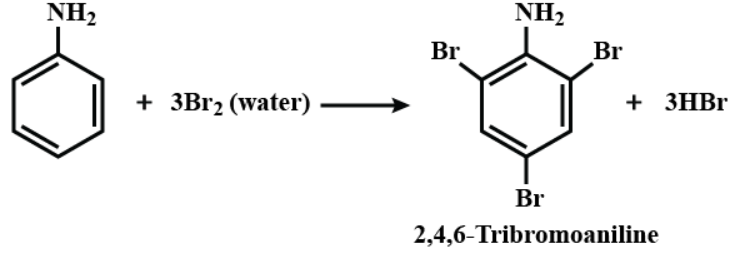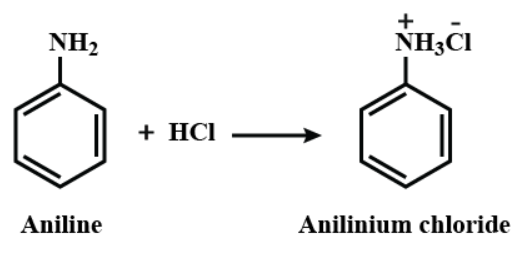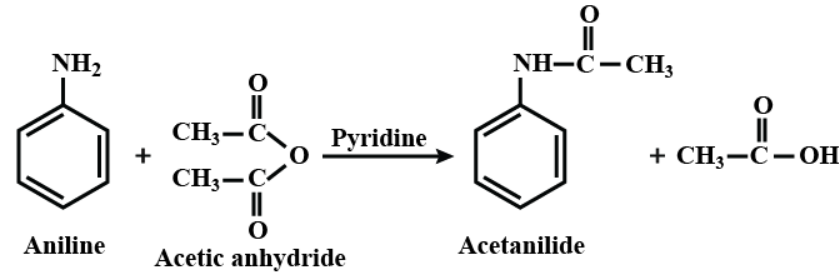(b) Alcohols have a higher boiling point than amines because oxygen, being more electronegative, forms stronger hydrogen bonds than nitrogen.
Tertiary amine has the lowest boiling point because there is no H present for hydrogen bonding.

Therefore the order is-

(CH3)3N < C2H5NH2 < C2H5OH

(c) When(CH3)2 – NH reacts with nitrous acid, an oily layer of N-nitrosamines forms that is insoluble in aqueous mineral acids. (CH3)3N, on the other hand, reacts with nitrous acid to form soluble nitrite salts with no ppt.

Question 14:

(a) Which poisonous gas is evolved when white phosphorus is heated with conc. NaOH solution? Write the chemical equation.

(b) Write the formula of the first noble gas compound prepared by N. Bartlett. What inspired N. Bartlett to prepare this compound?

(c) Fluorine is a stronger oxidizing agent than chlorine. Why?

(d) Write one use of chlorine gas.

(e) Complete the following equation : CaF2 + H2SO4

(a) The poisonous gas is evolved when white phosphorus is heated with conc. NaOH solution is phosphine.

P4 + 3NaOH + 3H2O → NaH2PO2 + PH3

(b) Xe[PtF6] is the first noble gas compound prepared by N. Bartlett.

Neil Bartlett observed a noble gas’s reaction. First, he made a red compound with the formula O2[PtF6]. He later discovered that the first ionisation enthalpy of molecular oxygen (1175 kJmol–1) was nearly identical to that of xenon (1170 kJmol–1). He attempted to make the same type of compound with Xe and was successful in making another red colour compound, Xe[PtF6]by combining PtF6 and xenon.

(c) The electrode potential of F2 and Cl2 are:

F2 + 2e → 2F ; E° = +2.87V

Cl2 + 2e→ 2Cl ; E° =1.36V

Since E° for F2/F is higher than that of Cl2/Cl, therefore, F2 is more easily reduced than Cl2. As a result, F2 is stronger oxidising agent than Cl2.

(d) Chlorine is used as a disinfectant.

(e) CaF2 + H2SO4 → 2HF + CaSO4

Question 15:

(a) Account for the following:

1. The bond angle in NH4+ is greater than that in NH3.
2. Reducing character decreases from SO2 to TeO2.
3. HClO3 is a stronger acid than HClO.

(b) Draw the structures of the following:

1. H2S2O8
2. XeOF4

(a)

1. The lone pair of electrons in NH3 strongly repel the bonded pair of electrons, reducing the bond angle. However, because NH4+ lacks a lone pair of electrons, there is no lone pair–bond pair repulsion. As a result, the bond angle in NH4+ is greater than that in NH3.
2. From SO2 to TeO2, the reducing character decreases because as the size of the central atom decreases, the electronegativity decreases and the metallic character increases.
3. The acid is much stronger if the number of oxygen atoms is more. In HClO3 and HClO2, HClO3 has more oxygen therefore it is more acidic.

(b)

1. The structure of H2S2O8 is-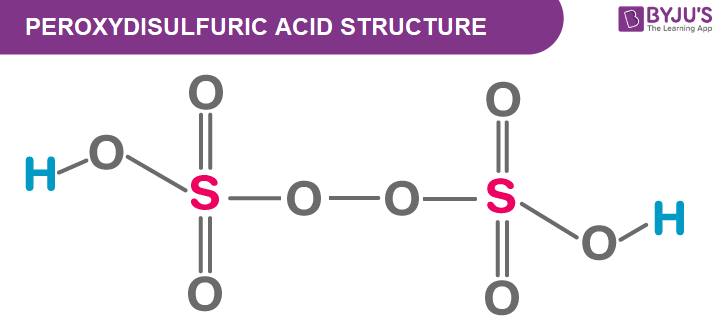2. The structure of XeOF4 is-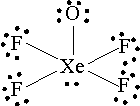Question 16:

(a) For a reaction A + B → P the rate is given by Rate = k [A] [B]2

1. How is the rate of reaction affected if the concentration of B is doubled?
2. What is the overall order of reaction if A is present in large excess?

(b) A first order reaction takes 23.1 minutes for 50% completion. Calculate the time required for 75% completion of this reaction. (Given : log 2 = 0.301, log 3 = 0.4771, log 4 = 0.6021)

(a) For a reaction A + B → P the rate is given by Rate i.e., R1= k [A] [B]2

1. If the concentration of B is doubled,
R2 = k [A] [2B]2
On dividing R1 and R2
R2 = 4R1
Therefore, the rate of reaction will become 4 times.
2. If A is present in large quantities, the rate of the reaction will be independent of A and will be determined solely by the concentration of B. The reaction’s overall rate will be 2.

(b)

$$\begin{array}{l}t_{1/2}=23.1min\end{array}$$

$$\begin{array}{l}t_{1/2}=\frac{0.693}{k}\end{array}$$

$$\begin{array}{l}k=\frac{0.693}{23.1}=0.03\end{array}$$

$$\begin{array}{l}k=\frac{2.303}{t}log\frac{\left [ R_{O} \right ]}{\left [ R \right ]}\end{array}$$

$$\begin{array}{l}k=\frac{2.303}{t}log\frac{\left [100 \right ]}{\left [ 25 \right ]}\end{array}$$

$$\begin{array}{l}k=\frac{2.303}{t}log4\end{array}$$

$$\begin{array}{l}t=\frac{2.303}{k}log4=\frac{2.303}{0.03}log4\end{array}$$

t = 46.22 min

Question 17:

(a) Write the chemical reaction involved in Etard reaction.

(b) Arrange the following in the increasing order of their reactivity towards nucleophilic addition reaction : CH3CHO, C6H5COCH3, CH3COCH3

(c) Why pKa of Cl–CH2–COOH is lower than the pKa of CH3COOH?

(d) Write the product in the following reaction.

$$\begin{array}{l}CH_{3}CH_{2}CH=CH-CH_{2}CN\xrightarrow[2.H_{2}O]{1.\left ( i-Bu \right )_{2}AlH}\end{array}$$

(e) A and B are two functional isomers of compound C3H6O. On heating with NaOH and I2, isomer A forms yellow precipitate of iodoform whereas isomer B does not form any precipitate. Write the formulae of A and B.

(a) The chemical reaction involved in Etard reaction is-(b) he correct order is CH3COCH3 < CH3CHO < C6H5COCH3

(c) Since -Cl is an electron withdrawing group, it stabilises the ClCH2COO anion and raises its pKa value. As a result, chloroacetic acid has a lower pKa value than acetic acid.

(d) The product in the following reaction is CH3CH=CHCHO.(e) A and B are two functional isomers of compound C3H6O. On heating with NaOH and I2, isomer A forms a yellow precipitate of iodoform whereas isomer B does not form any precipitate. The formulae of A is CH3–CO– CH3 that is Propanone and B is C2H5CHO that is Propanal.

Question 18:

For the hydrolysis of methyl acetate in aqueous solution, the following results were obtained :

 t/s 0 30 60 [CH3COOCH3]/mol L–1 0.6 0.3 0.15

(i) Show that it follows a pseudo first order reaction, as the concentration of water remains constant.

(ii) Calculate the average rate of reaction between the time interval 30 to 60 seconds.

(i)

$$\begin{array}{l}k=\frac{2.303}{t}log\frac{\left [ A \right ]_{0}}{A}\end{array}$$

Where, [A]0 is the initial concentration of reactant and [A] is the final concentration of reactant

At t1= 30 sec,

$$\begin{array}{l}k=\frac{2.303}{30}log\frac{0.60}{0.30}\end{array}$$

k1 = 0.07677 log 2

k1= 0.0231 sec–1

For t2 = 60 sec

$$\begin{array}{l}k=\frac{2.303}{60}log\frac{0.60}{0.15}\end{array}$$

$$\begin{array}{l}k=\frac{2.303}{60}log\left ( 2 \right )^{2}\end{array}$$

k2= 0.0231 sec–1

k1 = k2

Hence, the reaction is a pseudo first order reaction.

(ii) The average rate of reaction between the time interval 30 to 60 seconds-

$$\begin{array}{l}Rate=\frac{\Delta x}{\Delta t}=\frac{0.30-0.15}{60-30}=0.005 mol L^{-1}sec^{-1}\end{array}$$

Question 19:

The elements of 3d transition series are given as : Sc Ti V Cr Mn Fe Co Ni Cu Zn

(i) Write the element which is not regarded as a transition element. Give reason.

(ii) Which element has the highest m.p ?

(iii) Write the element which can show an oxidation state of +1.

(iv) Which element is a strong oxidizing agent in +3 oxidation state and why ?

(i) Zinc (Zn) is not considered a transition element because it lacks a partially filled d-orbital in either the ground state or the stable +2 oxidation state.

(ii) Cr, because Cr has configuration 3d54s1 , 6 unpaired electrons participated in formation of metallic bonds

(iii). Cu is the only metal in the first transition series (3d series) that frequently exhibits the +1 oxidation state. This is because Cu’s electronic configuration is 3d10 4s1, and losing one electron results in a stable 3d10 configuration.

(iv) Iron is a strong oxidizing agent in +3 oxidation state. It readily accepts an electron to form +2 oxidation state.
Iron in +3 oxidation state can also accept 3 electrons for 0 oxidation state.

Question 20:

(a) Account for the following :

1. Mn shows the highest oxidation state of +7 with oxygen but with fluorine it shows the highest oxidation state of +4
2. Zirconium and Hafnium exhibit similar properties.
3. Transition metals act as catalysts.

(b) Complete the following equations :

1. $$\begin{array}{l}2MnO_{2}+4KOH+O_{2}\xrightarrow[]{\Delta }\end{array}$$
2. $$\begin{array}{l}Cr_{2}O_{7}^{2-}+14H^{+}+6I^{-}\to \end{array}$$

(a)

1. Mn does not exhibit +7 oxidation with fluorine because fluorine cannot form multiple bonds with Mn. If seven fluorine atoms are attracted to Mn, the δ+ at Mn will be too high, resulting in an unstable compound.
2. Zirconium and Hafnium have similar properties because the atomic radii of elements in 5d decrease overall due to imperfect shielding of f electrons, which is unable to counterbalance the effect of increased nuclear charge.
3. Due to their ability to exhibit multiple oxidation states and their proclivity to form complexes, transition metals act as catalysts.

(b)

1. $$\begin{array}{l}2MnO_{2}+4KOH+O_{2}\xrightarrow[]{\Delta }2K_{2}MnO_{4}+2H_{2}O\end{array}$$
2. $$\begin{array}{l}Cr_{2}O_{7}^{2-}+14H^{+}+6I^{-}\to 2Cr^{3+}+3I_{2}+7H_{2}O\end{array}$$

Question 21:

(a) The conductivity of 0.001 mol L-1 solution of CH3COOH is 3.905 × 10-5 S cm-1. Calculate its molar conductivity and degree of dissociation(α).

Given λº(H+ ) = 349.65 cm2 mol–1 & λº(CH3COO ) = 40.95 cm2 mol-1

(b) Define electrochemical cell. What happens if external potential applied becomes greater than Eºcell of electrochemical cell ?

(a) The conductivity k = 3.905 × 10-5 S cm-1, Concentration C = 0.001M

The molar conductivity at a given solution is

$$\begin{array}{l}\Lambda =\frac{1000k}{C}\end{array}$$

$$\begin{array}{l}\Lambda =\frac{1000\times 3.905\times 10^{-5}}{0.001}=39.05 S cm^{2}mol^{-1}\end{array}$$

At infinite dilution,

$$\begin{array}{l}\Lambda _{0}=\Lambda _{H^{+}}+\Lambda _{CH_{3}COO^{-}}\end{array}$$

Λ0 = 34.96 + 40.9 = 390.5 Scm2/mol

The degree of disscociation,

$$\begin{array}{l}\alpha = \frac{\Lambda }{\Lambda _{0}}=\frac{39.05}{390.5}=0.1\end{array}$$

(b) An electrochemical cell is a device that uses electricity to study chemical reactions. It is made up of two electronic conductors, such as metal plates or carbon rods, that are immersed in an electrolytic or ionic conductor, such as an aqueous electrolyte solution or a pure electrolyte liquid.

Thus, an electrochemical cell is a device that converts chemical energy into electrical energy by decreasing free energy during an indirect redox reaction.

When the applied external potential exceeds the Eºcell of an electrochemical cell, the electrochemical cell behaves as an electrolytic cell. All chemical reactions will be reversed, and electrical energy converted into chemical energy.

Question 22:

(a) Calculate Eºcell for the following reaction at 298 K:

2Cr(s) + 3Fe2+ (0.01 M) → 2Cr3+ (0.01 M) + 3Fe(s)

Given : Ecell = 0.261 V

(b) Using the Eº values of A and B, predict which one is better for coating the surface of iron [Eº(Fe2+/Fe) = –0.44 V] to prevent corrosion and why ?

Given : Eº(A2+/A) = –2.37 V : Eº(B2+/B) = –0.14 V

(a) 2Cr(s) + 3Fe2+ (0.01 M) → 2Cr3+ (0.01 M) + 3Fe(s)

Given : Ecell = 0.261 V

$$\begin{array}{l}E_{cell}=E^{\circ}_{cell}-\frac{0.059}{n}log\frac{\left [ product \right ]}{\left [ reactant \right ]}\end{array}$$

$$\begin{array}{l}E^{\circ}_{cell}= E_{cell}+\frac{0.059}{n}log\frac{\left [ product \right ]}{\left [ reactant \right ]}\end{array}$$

$$\begin{array}{l}E^{\circ}_{cell}=0.0261+\frac{0.059}{6}log\frac{\left [ Cr^{3+} \right ]^{2}}{\left [ Fe^{2+} \right ]^{3}}\end{array}$$

$$\begin{array}{l}E^{\circ}_{cell}=0.0261+\frac{0.059}{6}log\frac{\left [ 10^{-2} \right ]^{2}}{\left [ 10^{-2} \right ]^{3}}\end{array}$$

$$\begin{array}{l}E^{\circ}_{cell}=0.0261+\frac{0.059}{6}log10^{2}\end{array}$$

$$\begin{array}{l}E^{\circ}_{cell}=0.0261+\frac{0.059\times 2}{6}\end{array}$$

$$\begin{array}{l}E^{\circ}_{cell}=0.0261+0.01966=0.28068 V \approx 0.281 V\end{array}$$

(b) The corrosion is an oxidation of iron phenomenon, it is critical to consider the oxidation potentials of all elements. Element with a higher oxidation potential than Fe will oxidise faster than iron, preventing corrosion in iron.

Oxidation potential of Fe = -0.44 V

Oxidation potential of A = -2.37 V

Oxidation potential of B = -0.14 V

Since A has a higher oxidation potential than iron, it can be used to coat the iron’s surface.

Question 23:

(a) Write the reactions involved in the following :

(i) Etard reaction

(ii) Stephen reduction

(b) How will you convert the following in not more than two steps :

(i) Benzoic acid to Benzaldehyde

(ii) Acetophenone to Benzoic acid

(iii) Ethanoic acid to 2-Hydroxyethanoic acid

(a)

(i) Etard reaction(ii) The reaction describes the preparation of aldehydes from nitriles with the help of tin(II) chloride and hydrochloric acid and the quenching of the resulting iminium salt with water. Ammonium chloride is another useful byproduct produced by this reaction.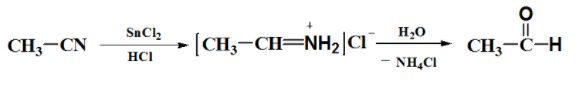(b)

(i) Benzoic acid to Benzaldehyde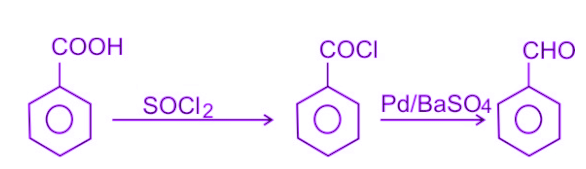(ii) Acetophenone to Benzoic acid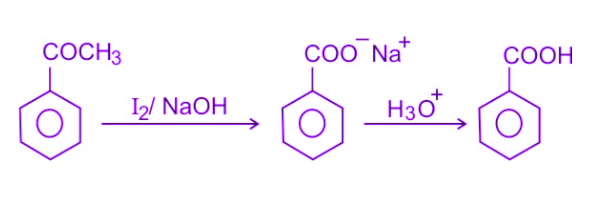(iii) Ethanoic acid to 2-Hydroxyethanoic acid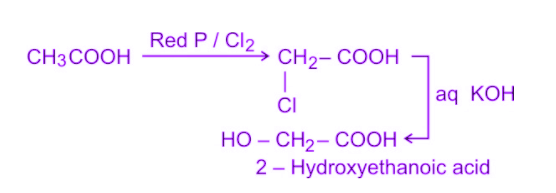Question 24:

(a) 30 g of urea (M = 60 g mol-1) is dissolved in 846 g of water. Calculate the vapour pressure of water for this solution if the vapour pressure of pure water at 298 K is 23.8 mm Hg.

(b) Write two differences between ideal solutions and non-ideal solutions.

$$\begin{array}{l}\frac{P_{A}^{\circ }-P_{A}}{P_{A}^{\circ }}=\frac{w_{B}\times M_{A}}{M_{B}\times w_{A}}\end{array}$$

$$\begin{array}{l}\frac{23.8-P_{A}}{23.8}=\frac{30\times 18}{60\times 846}\end{array}$$

23.8 – PA = 23.8 × [(30 × 18)/(60 × 546)] = 0.2532

PA = 23.55 mm Hg

(b) Two differences between ideal solutions and non-ideal solutions-

 Ideal solution Non ideal solution It follows Raoult’s law throughout the entire concentration range. It violates Raoult’s law. The total vapour pressure of a solution is the sum of the vapour pressures of its constituents. The sum of the vapour pressures of the individual components does not equal the total vapour pressure of the solution.

Question 25:

(a) Write the products formed when CH3CHO reacts with the following reagents :

(i) HCN

(ii) H2N – OH

(iii) CH3CHO in the presence of dilute NaOH

(b) Give simple chemical tests to distinguish between the following Pairs of compounds.

(i) Benzoic acid and Phenol

(ii) Propanal and Propanone.

(a)

(i) Acetaldehyde (CH3CHO) reacts with hydrogen cyaide HCN to give 2-hydroxypropapanenitrile as product.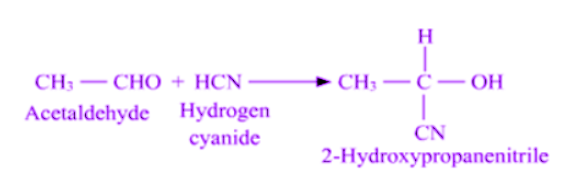(ii) Acetaldehyde (CH3CHO) reacts with Hydroxylamine (NH2OH) to give acetaldoxime as a product.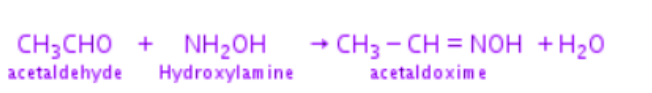(iii) The type of Aldol reaction that produced 3-hydroxybutanal is the reaction of acetaldehyde with acetaldehyde in the presence of dilute NaOH. When the reaction is continued with heat, the aldol condensation product, But-2-enal, is produced.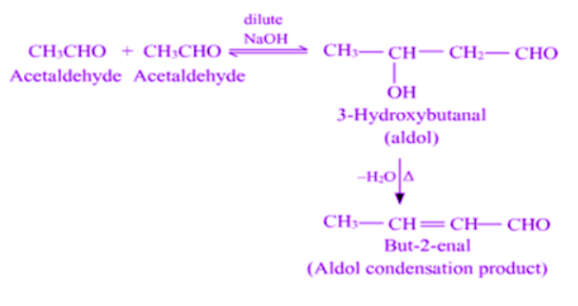(b) Chemical tests to distinguish between the following Pairs of compounds-

(i) Benzoic acid and phenol- These can be distinguished by FeCl3 tests. Phenol reacts with FeCl3 to give violet colour, whereas benzoic acid gives buff coloured precipitate.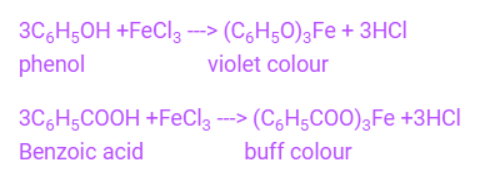(ii) Propanal and propanone: They are distinguished by the iodoform test. When propanol reacts with I2 in the presence of NaOH it does not gives iodoform test, whereas propanone gives the iodoform test when it reacts with I2 in the presence of NaOH.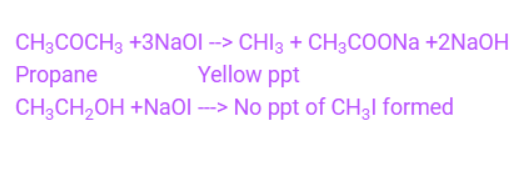Question 26:

(a) A 10% solution (by mass) of sucrose in water has a freezing point of 269.15 K. Calculate the freezing point of 10% glucose in water if the freezing point of pure water is 273.15 K.

Given : (Molar mass of sucrose = 342 g mol-1) (Molar mass of glucose = 180 g mol-1)

(b) Define the following terms :

(i) Molality (m)

(ii) Abnormal molar mass

(a) Here,

Molar mass of sucrose = 342 g mol–1

Molar mass of glucose = 180 g mol–1

ΔTf = Kf m

Here,

$$\begin{array}{l}m = \frac{w_{2}\times 1000}{M_{2}\times M_{1}}\end{array}$$

$$\begin{array}{l}273.15-269.15=\frac{k_{f}\times 10\times 1000}{342\times 90}\end{array}$$

$$\begin{array}{l}k_{f}=12.3K\frac{kg}{mol}\end{array}$$

ΔTf = Kf m

$$\begin{array}{l}=\frac{12.3\times 10\times 1000}{180\times 90}\end{array}$$

= 7.6 K

Tf = 273.15 – 7.6 = 365.55 K

(b)

(i) Molality (m) is the number of moles of solute present per kg of solvent:

$$\begin{array}{l}m=\frac{n}{W}\end{array}$$

(ii) When molar masses are calculated, abnormal molar masses occur if they are greater or less than the expected value. The colligative properties are used to calculate these.

$$\begin{array}{l}i=\frac{Observed Molecular Mass}{Theoretical Molecular Mass}\end{array}$$

Question 27:

(a) Following are the transition metal ions of 3d series :

Ti4+, V2+, Mn3+, Cr3+

(Atomic numbers : Ti = 22, V = 23, Mn = 25, Cr = 24)

(i) Which ion is most stable in an aqueous solution and why ?

(ii) Which ion is a strong oxidising agent and why ?

(iii) Which ion is colourless and why ?

(b) Complete the following equations:

(i)

$$\begin{array}{l}2MnO_{4}^{-}+16H^{+}+5S^{2-}\to \end{array}$$

(ii)

$$\begin{array}{l}KMnO_{4}\xrightarrow[]{heat}\end{array}$$

(i) Ti4+ is most stable as it has completely filled 3p orbital.

(ii) Mn3+ is the strongest oxidising agent as it requires only one electron to half fill its 3d orbital

(iii)Ti4+ will be colorless due to its empty 3d orbital.

(b) Complete the following equations:

(i)

$$\begin{array}{l}2MnO_{4}^{-}+16H^{+}+5S^{2-}\to 2Mn^{2+}+5S+5H_{2}O\end{array}$$

(ii)

$$\begin{array}{l}2KMnO_{4}\xrightarrow[]{heat}K_{2}MnO_{4}+MnO_{2}+O_{2}\end{array}$$

Question 28:

a) Write the reactions involved in the following :

1. Etard reaction2. Stephen reductionb)How will you convert the following in not more than two steps :

1. Benzoic acid to Benzaldehyde
2. Acetophenone to Benzoic acid
3. Ethanoic acid to 2-Hydroxyethanoic acid

(a)

1. Etard reaction
2. The reaction describes the preparation of aldehydes from nitriles with the help of tin(II) chloride and hydrochloric acid and the quenching of the resulting iminium salt with water. Ammonium chloride is another useful by product produced by this reaction.

(b)

1. Benzoic acid to Benzaldehyde2. Acetophenone to Benzoic acid3. Ethanoic acid to 2-Hydroxyethanoic acidQuestion 29:

(a) 30 g of urea (M = 60 g mol-1) is dissolved in 846 g of water. Calculate the vapour pressure of water for this solution if vapour pressure of pure water at 298 K is 23·8 mm Hg.

(b) Write two differences between ideal solutions and non-ideal solutions.

(a)

$$\begin{array}{l}\frac{23.8-P_{A}}{23.8}=\frac{30\times 18}{60\times 846}\end{array}$$

23.8 – PA = 23.8 × [(30 × 18)/(60 × 546)] = 0.2532

PA = 23.55 mm Hg

(b) Two differences between ideal solutions and non-ideal solutions.

Question 29:

(a) State Raoult’s law for a solution containing volatile components. How does Raoult’s law become a special case of Henry’s law ?

(b) 1.00 g of a non-electrolyte solute dissolved in 50 g of benzene lowered the freezing point of benzene by 0.40 K. Find the molar mass of the solute. (Kf for benzene = 5.12 K kg mol–1)

(a) The partial vapour pressure of a liquid component in solution is directly proportional to its mole fraction. The partial pressure of the volatile component or gas in solution is directly proportional to its mole fraction. The proportionality constant KH is the only one that differs from PA°. Raoult’s law thus becomes a special case of Henry’s law in which Ks equals PA°.

(b)

$$\begin{array}{l}\Delta T_{f}=K_{f}m\end{array}$$

$$\begin{array}{l}\Delta T_{f}=K_{f}\frac{W_{b}\times 1000}{M_{b}\times W_{a}}\end{array}$$

$$\begin{array}{l}0.40=5.12\frac{1\times 1000}{M_{b}\times 50}\end{array}$$

Mb = 256 g/mol.

Test your Knowledge on Important 5 marks questions for cbse class 12 chemistry!